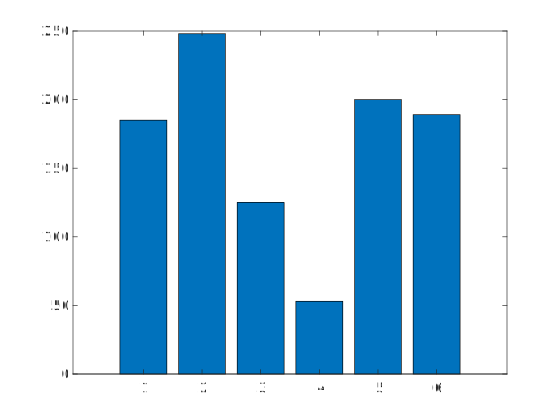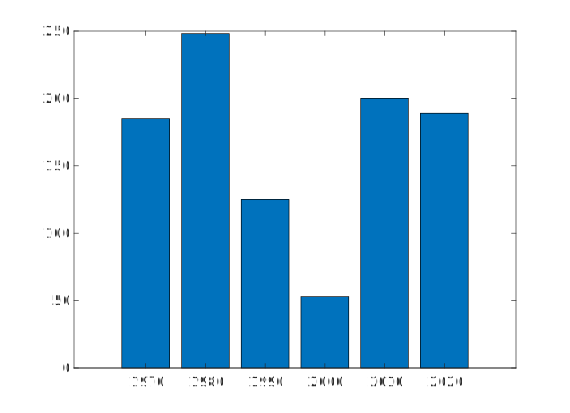👋 Hello! I'm Alphonsio the robot. Ask me a question, I'll try to answer.

In Matlab, how to draw a bar graph?

The MATLAB ﻿bar() function creates a bar graph in a figure. Let's consider the following vectors :

x = [1970 1980 1990 2000 2010 2020];
y = [185  248  125  53   200  189];

The following creates a bar graph with one bar for each element of ﻿y:

﻿bar (y)The following draws the bars at the location specified by ﻿x:

bar(x,y);More